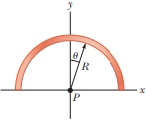# A line of positive charge is formed into a semicircle of radius R = 60.0 cm as shown in Figure P23.41. The charge per unit length along the semicircle is given by the expression λ = λ 0 cos θ . The total charge on the semicircle is 12.0 μ C. Calculate the total total on a charge of 3.00 μ C placed at the center of curvature P . Figure P23.41### Physics for Scientists and Enginee...

10th Edition
Raymond A. Serway + 1 other
Publisher: Cengage Learning
ISBN: 9781337553278

#### Solutions

Chapter
Section### Physics for Scientists and Enginee...

10th Edition
Raymond A. Serway + 1 other
Publisher: Cengage Learning
ISBN: 9781337553278
Chapter 23, Problem 41AP
Textbook Problem
1108 views

## A line of positive charge is formed into a semicircle of radius R = 60.0 cm as shown in Figure P23.41. The charge per unit length along the semicircle is given by the expression λ = λ0 cos θ. The total charge on the semicircle is 12.0 μC. Calculate the total total on a charge of 3.00 μC placed at the center of curvature P.Figure P23.41To determine

The net force on a charge placed at the center of the curvature.

### Explanation of Solution

The radius of the semicircle is 60.0cm, the expression for the charge per unit area is λ=λ0cosθ and the total charge on the semicircle is 12.0μC.

Write the expression for the component of electric field along x axis

dE=dEcosθ=(KdQR2)cosθ                                                                                      (I)

Here, dQ is a segment of charge on the semicircle, dE is electric field due to a segment of charge, θ is the angle between the line joining the point and the charge with the vertical, K is the coulomb’s constant and R is the radius of the semicircle.

Write the expression for segmental arc length

dl=Rdθ

Here, dl is the arc length of the segment.

Write the expression for the total charge on the semicircle

dQ=λdlQ=π2π2λdl

Here, Q is the net charge on the semicircle and λ is the linear charge density.

Substitute λ0cosθ for λ and Rdθ for dl in above equation.

Q=π2π2λ0cosθRdθ=[λ0Rsinθ]π2π2=λ0(2R)λ0=Q2R

Substitute λdl for dQ, λ0cosθ for λ and Rdθ for dl in equation (I).

dEy=(KλdlR2)cosθ=(Kλ0cosθRdθR2)cosθ=(Kλ0R)cos2θdθ

Integrate the above equation to find the total electric field

E=π2π2dE=π2π2(K

### Still sussing out bartleby?

Check out a sample textbook solution.

See a sample solution

#### The Solution to Your Study Problems

Bartleby provides explanations to thousands of textbook problems written by our experts, many with advanced degrees!

Get Started

Find more solutions based on key concepts
Under certain circumstances, amino acids can be converted to glucose and so serve the energy needs of the brain...

Nutrition: Concepts and Controversies - Standalone book (MindTap Course List)

What do enzymes do in metabolic reactions?

Human Biology (MindTap Course List)

True or false? DNA barcoding can identify an individual as belonging to a particular species.

Biology: The Unity and Diversity of Life (MindTap Course List)